Refer to our Texas Go Math Grade 2 Answer Key Pdf to score good marks in the exams. Test yourself by practicing the problems from Texas Go Math Grade 2 Lesson 12.1 Answer Key Add Equal Groups.

Explore

Draw to model the problem. Write the skip counting to find the total.
Jim’s shoes__________ shoes
Ella’s flower petals__________ flower petals

FOR THE TEACHER • Read this problem to children. Then have them draw a model and skip count to find the answer. Jim has 4 pairs of shoes. How many shoes does he have? Repeat for the following problem. Ella has 5 flowers. Each flower has 5 petals. How many petals are there?
Jim has 4 pairs of shoes.
each pair has 2 shoes
4 x 2 = 8
Ella has 5 flowers.
Each flower has 5 petals.
5 x 5 = 25
25 petals are there
Explanation:
8  shoes
25 petals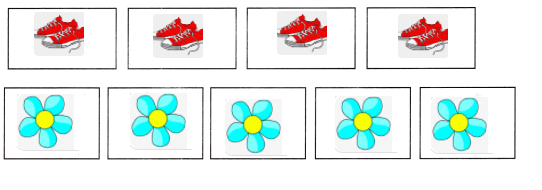Math Talk
Mathematical Processes

Explain how skip counting helped you solve the second problem.
In 2 cases skip counting is used
in the way of multiplication.

Model and Draw

Chen has 4 pages of postcards. Each page has 5 postcards. How many postcards does Chen have?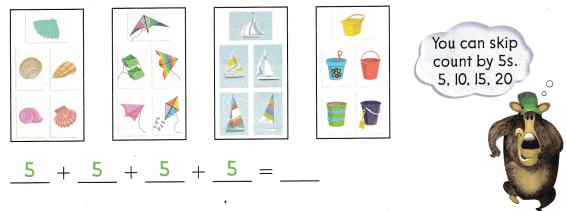Chen has ___________ postcards.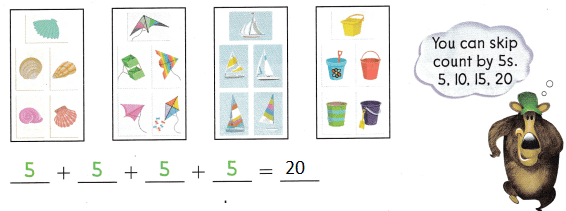Explanation:
5 x 4 = 20
total there are 20 postcards

Share and Show

Add equal groups to find the total number of objects.

Question 1.
3 bowls of 3 apples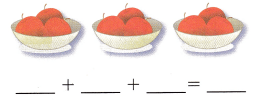________ apples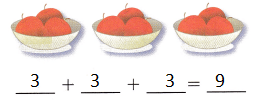Explanation:
The sum is found with the help of images

Question 2.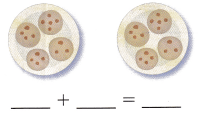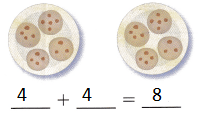2 x 4 = 8
Explanation:
The sum is found with the help of images

Question 3.
3 bags of 5 potatoes__________ potatoes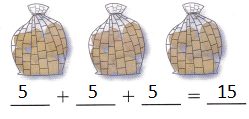15 potatoes
3 x 5 = 15
Explanation:
The sum is found with the help of images

Question 4.
2 boxes of 8 oranges__________ oranges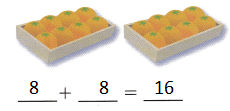16 oranges
2 x 8 = 16
Explanation:
The sum is found with the help of images

Problem Solving

Use counters. Draw and then add equal groups. Find the total.

Question 5.
5 groups of 4 counters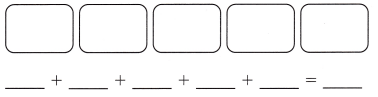___________ countersExplanation:
4 Balloons are shared among 5 friends
4 x 5 = 20

Question 6.
4 groups of 3 counters___________ counters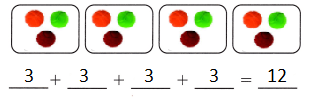Explanation:
3 x 4 = 12
12 balls shared among 4 groups
each group has 3 balls

Question 7.
H.O.T. Multi-Step Jeff has 5 toy cars. Then his sister gives him 3 more toy cars. Each toy car has 4 wheels. How many wheels are on Jeff’s toy cars?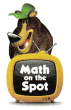_________ wheels
2 wheels
Explanation:
Jeff has 5 toy cars.
Then his sister gives him 3 more toy cars.
Each toy car has 4 wheels.
5 + 3 = 8
8 ÷ 4 = 2
2 wheels are on Jeff’s toy cars

Question 8.
H.O.T. Angela used these counters to act out a problem.Write a problem that Angela could have solved with these counters.
Angela went picnic with her friends
they were 8 friends
And 4 tents
How many friends should she accommodate for each tent
Explanation:
8 ÷ 4 = 2

Question 9.
Analyze There are 2 juice boxes for each person at a picnic. There are 10 people at the picnic. How many juice boxes are there?
(A) 12
(B) 8
(C) 20
Explanation:
There are 2 juice boxes for each person at a picnic.
There are 10 people at the picnic.
10 x 2 = 20
20 juice boxes are there

Question 10.
Use counters to model 3 equal groups of 5 objects. Draw a picture of the groups below. Then write an addition sentence for your model.
_________ + _________ + _________ = _________
________ objects
5 + 5 + 5 = 15
15 objects
Explanation:Question 11.
TEXAS Test Prep Brett has 3 bags of shells. He has 4 shells in each bag. How many shells does he have?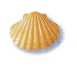(A) 12
(B) 7
(C) 16
Explanation:
Brett has 3 bags of shells.
He has 4 shells in each bag.
3 x 4 = 12
12 shells that he have

TAKE HOME ACTIVITY • Ask your child to show how he or she solved on exercise in the lesson.
2 boxes of 3 toy tops_________ + _________
_________ toy tops
3 + 3
6 toy tops
Explanation:
The sum is found with the help of images
Explanation:
Addition is done in the above problem

### Texas Go Math Grade 2 Lesson 12.1 Homework and Practice Answer Key

Add equal groups to find the total number of objects.

Question 1.
2 boxes of 3 toy tops_________ + _________
_________ toy tops
3 + 3
6 toy tops
Explanation:
The sum is found with the help of images

Question 2.
4 groups of 5 pennies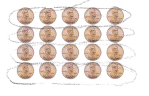_________ + _________ + _________ + _________ = _________
_________ pennies
5 + 5 + 5 + 5 = 20
20 pennies
Explanation:
The sum is found with the help of images

Question 3.
3 groups of 4 counters_________ + _________ + _________ = _________
_________ counters
4 + 4 + 4 = 12
12 counters
Explanation:
The sum is found with the help of images

Problem Solving

Solve. Write or draw to explain.

Question 4.
Multi-Step Jonathan has 2 toy cars. Then he buys 2 more toy cars. Each toy car has 4 wheels. How many wheels are on Jonathan’s toy cars?
___________ wheels
16 wheels
Explanation:
Jonathan has 2 toy cars.
Then he buys 2 more toy cars.
Each toy car has 4 wheels.
2 + 2 = 4
4 x 4 = 16
16 wheels are on Jonathan’s toy cars

Lesson Check

Question 5.
Linda has 6 bags of ribbons. She has 5 ribbons in each bag. How many ribbons does she have?
(A) 25
(B) 30
(C) 11
Explanation:
Linda has 6 bags of ribbons.
She has 5 ribbons in each bag.
6 x 5 = 30
30 ribbons that she have

Question 6.
Jason’s family is having a cookout. His father plans to cook 3 small pieces of chicken for each person. How many pieces of chicken will he cook for 7 people?
(A) 21
(B) 10
(C) 24
Explanation:
Jason’s family is having a cookout.
His father plans to cook 3 small pieces of chicken for each person.
3 x 7 = 21
21 pieces of chicken that  he cook for 7 people

Question 7.
Julie is having a dinner party. She wants to have 2 bottles of water for each person. There will be a total of 9 people at dinner. How many bottles of water does she need?
(A) 20
(B) 16
(C) 18
Explanation:
Julie is having a dinner party. She wants to have 2 bottles of water for each person.
There will be a total of 9 people at dinner.
9 x 2 = 18
18 bottles of water that she need

Question 8.
Mark, his sister, and his 4 friends are sharing a box of mini pizzas. Each person will get 5 mini pizzas. What is the total number of mini pizzas in the box?
(A) 20
(B) 30
(C) 25